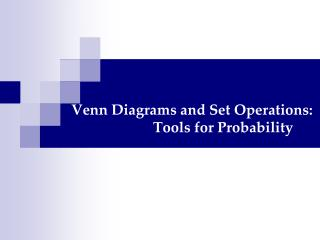DownloadDownload PresentationVenn Diagrams and Set Operations: Tools for Probability

Venn Diagrams and Set Operations: Tools for Probability

Télécharger la présentationVenn Diagrams and Set Operations: Tools for Probability

- - - - - - - - - - - - - - - - - - - - - - - - - - - E N D - - - - - - - - - - - - - - - - - - - - - - - - - - -
Presentation Transcript

1. Properties of the Probability of an Event • Here are some properties of the probability of an event that everyone must remember. • Let E be an event of a sample space S. • If E is the empty set, then P(E)=0. • For instance, if two dice are tossed, the probability that the sum of the faces that turn up is less than 2 is 0. • If E is the whole sample space S, then P(E)=1. • For instance, if two dice are tossed, the probability that the sum of the faces that turn up is between 2 and 12, inclusive, is 1. • Otherwise, 0<P(E)<1. • That is, the probability of an event is always positive and is never more than 1.

2. Sets and Venn diagrams can help us investigate other interesting properties of the probability of an event.

3. Example #1 • 12,000 people voted for a politician in his first election, 15,000 voted for him in his second, and 3,000 voted for him in both elections. 55,000 people voted in the elections. • What is the probability that a randomly chosen voter voted for the politician • in at least 1 one of the elections? • in neither one of the elections?

4. We can extend the use of Venn diagrams by filling them with probabilities of events in a sample space S. • But now, all the probabilities in the Venn diagram must add up to 1.

5. Example #2 • Let A and B be two events of a sample space S such that p(A)=0.45, p(B)=0.35, and p(A∩B)=0.15. • Use the given information to determine: • p(AUB) • p(A’∩B) • p(A’∩B’)

6. Venn diagrams can be quite helpful in solving real-life probability problems, as the next example shows.

7. Example #3 • The manager of a repair shop has observed that a car will require a tune-up with a probability of 0.6, a brake job with a probability of 0.1, and both with a probability of 0.02. • What is the probability that a car will require • either a tune-up or a brake job? • a tune-up but not a brake job? • neither type of repair?

8. The Complementary Rule If the probability of getting your dream job by age 30 is 0.25, then the probability of not getting it by that age is 0.75 • Thus if E is an event of a sample space S, then • p(E)=1-p(E’) • p(E’)=1-p(E) These results are referred to as the Complementary Rule

9. Example #4 • A bin in a bargain outlet contains 100 blank cassette tapes, of which 15 are known to be defective. • If a customer selects 20 of the tapes, determine the probability that at least 1 of them is defective.

10. The Complementary Rule that we have encountered is used in a memorable mathematics problem: The Birthday Problem